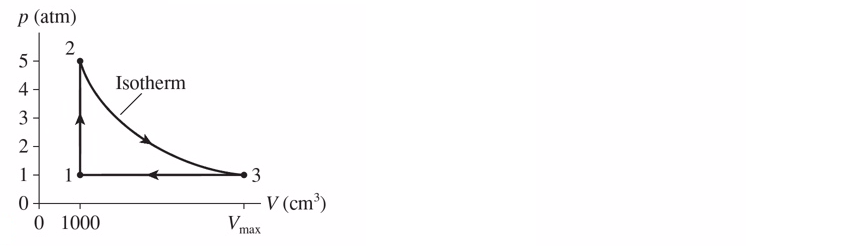# Problem: A heat engine uses 1.3 g of hydrogen and follows the cycle shown in the figure below. a) Find the pressure, volume, and temperature of the hydrogen at points 1, 2 and 3.b) What is the thermal efficiency of this engine?

95% (34 ratings)
###### Problem Details

A heat engine uses 1.3 g of hydrogen and follows the cycle shown in the figure below.

a) Find the pressure, volume, and temperature of the hydrogen at points 1, 2 and 3.

b) What is the thermal efficiency of this engine?Frequently Asked Questions

What scientific concept do you need to know in order to solve this problem?

Our tutors have indicated that to solve this problem you will need to apply the Heat Engines & PV Diagrams concept. You can view video lessons to learn Heat Engines & PV Diagrams. Or if you need more Heat Engines & PV Diagrams practice, you can also practice Heat Engines & PV Diagrams practice problems.